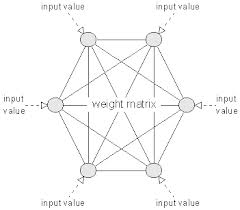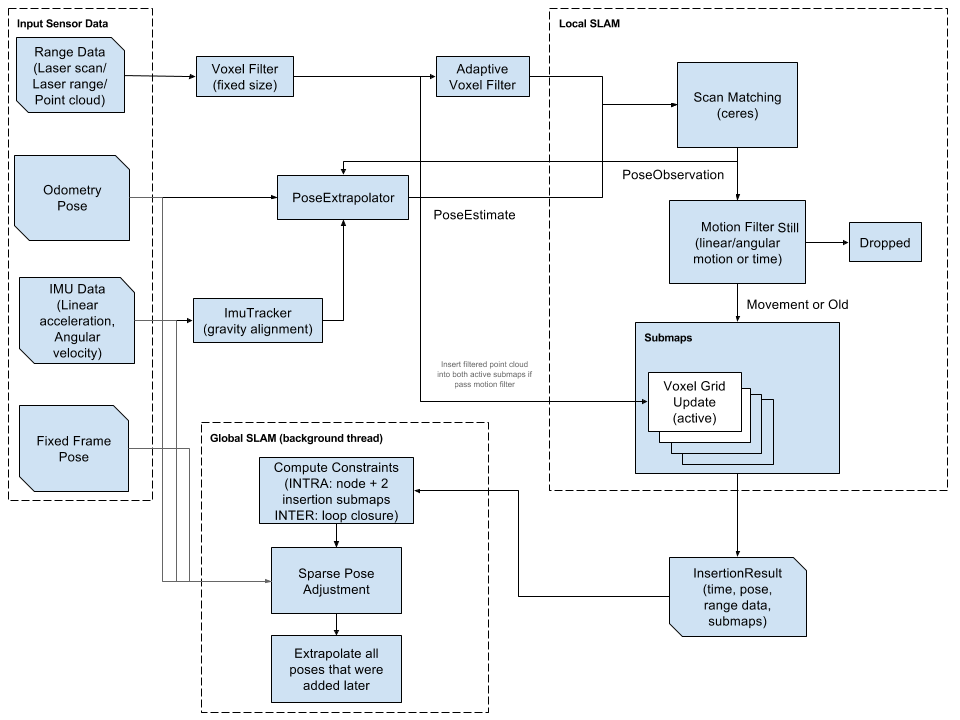Monday, April 7, 2014

Particle Swarm Optimization

Since my last post, I have spent a lot of time learning about biologically inspired optimization techniques.  Some commonly used biological machine learning methods include genetic algorithms and neural networks.  However, I decided to dive a little bit deeper and try out a few newer algorithms.  The two algorithms I have been working with are called Particle Swarm Optimization (PSO) and Ant Colony Optimization (ACO).  In this post I will talk about PSO.

Particle Swarm OptimizationThis is a representation of Particle Swarm Optimization.  The solution that is found for a particle is swayed by the global best and the individual particle's best solutions.  http://www.sciencepubco.com/index.php/JACST/article/view/84

PSO is a stochastic technique that is based on the way that swarms move towards a goal.  Each particle is a potential solution (or bee in a swarm) and it moves in some direction with a velocity.  The velocity is determined by a combination of the individual particle's best solution, the global swarm's best solution and an inertia weight.  First developed by Kennedy and Eberhart in 1995, each particle is updated with the equations shown below:http://web.ist.utl.pt/gdgp/VA/pso.htm
The interesting equation above is the velocity update.  Essentially, the new velocity for particle i is the addition of the previous velocity for that particle times the inertia weight (a function of how many iterations we have performed), the randomly weighted difference between the particle and the particle best and the randomly weighted difference between the particle and the global best.  randomly uniform weighting of the differences is a way of introducing unpredictability into the equation so that the system does not get stuck at a local minimum.  The position update just adds the new velocity to the previous position.

The algorithm for performing these actions is fairly straight-forward as well.  The idea is to update each particle and determine if that particle has a better fitness value than it has ever had before, and then determine if it has a better fitness than the swarm's best fitness.  This way, each particle has the equivalent of a unique mind as well as the swarm mind.  Below is the algorithm specifics:

1. A set of N particles are created such that each particle contains random values in the set.
2. It is determined whether each particle is its best case, if it is, particle i's best is this particle.
3. It is determined whether the best particle is the swarms best case, if it is, the swarm best is this particle.
4. Each particle's velocity and position are updated simultaneously with the new particle and swarm bests.
5. If the global best meets the termination condition or the max iterations are reached, stop.  Else, go back to step 2 and repeat.
Using this algorithm, I was able to solve the N-queens problem.  My implementation can be found here, as a new Seeker in the Do-Anything-Machine.  In the N-queens problem, each particle is an array of rows containing a number that corresponds to a column for the position of each queen.  (For an explanation of the N-queens problem, see the previous post).  The fitness function is simply the number of queens that are on the same horizontals, verticals and diagonals of the chess board.  If the fitness of a particle is zero, the solution is correct.

One issue that I have noticed is that it can usually find a local minimum of the system, but does not always find a global minimum.  My solution was to repeat the algorithm multiple times, until the system met the fitness requirement.

Feel free to let me know what you think of my work in the comments.  I am happy to provide links and help to those who are interested.

Monday, March 24, 2014

The Do-Anything-Machine and the Hopfield Neural Network

Machine learning has become a new fascination for me in the past few months.  I have delved into the complicated world of solving problems with neural nets, genetic algorithms and clustering techniques.  Recently, I started work on the Do-Anything-Machine, a project started by Eric Neuman to build an artificial intelligence for solving any type of problem.  This is clearly a huge task, so it has been open-sourced.  To get involved, I have developed this method of solving the N-Queens problem.

The problem states that there are a number of ways to place N number of Queens onto a chess board with NxN squares, such that none of the queens can capture each other.  One way of solving this problem is by using a Hopfield Neural Network configuration.  A Hopfield net is a single-layer network in which all of the neurons are connected.  In our case, each neuron represents a square on the board, so there are N x N neurons.  Each neuron connection is weighted in a way that all of diagonal, horizontal and vertical squares from the initial square are added into that neuron, and the rest are weighted with zero.   The inputs are a random binary array, where each value in the array is an input into one of the neurons.  The output is also a binary array, with each value being an output from one of the neurons.This is an example of a Hopfield network with 6 neurons. (http://www.nnwj.de/hopfield-net.html)
The general method has two parts:
- The Internal process
- The External process

The internal process is an asynchronous method of changing the output of each neuron.
1. A random neuron is picked
2. The input potential of the neuron is calculated by summing the values of all of the horizontal, vertical, and diagonal queens from that square
3. This gives a value that is fed through a sigmoid function
4. The resulting output is then fed back as an input
5. The process repeats

The External process is performed after N x N iterations of the internal process.
1. The internal process is performed N x N times so that the inputs have a chance to evenly distribute
2. The energy (or cost function) of the entire board is calculated
3. If after 3 External iterations, the change in energy is zero, then the system has stabilized, and you have reached local minima
4. If it has not stabilized, then repeat the external process

Due to the amount of randomness, the system converges in anywhere from 3-25 external iterations.  The code for the initial implementation is here.  Please check it out and join in on the project!

Working with Google's Cartographer SLAM Package

At my current position, at Canvas Construction, I have worked with a number of SLAM and localization packages. In the past few years, my wor...